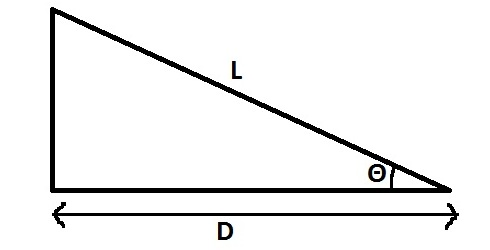# Tape Correction

The tape corrections used in surveying are as follows :

• Correction for Standardization.
• Correction for Slope.
• Correction of Pull.
• Correction for Temperature.
• Correction for Sag.
• Normal Tension.
• Correction for Misalignment.
• Reduction of length to mean sea level.

### Tape Correction for Standardization :

Correction per tape length (C) = l’ – l

Here,
l = nominal length of tape.
l’ = actual length of tape.

The correction is positive when the actual length is greater than the nominal length and vice versa. Total correction in the measured distance is given as :

### Correction for Slope (Always Negative):

Cs = L – D = L (1 – cos Θ) = 2L sin²Θ / 2 (-)Where,
D = horizontal equivalent
L = slope distance
Θ = angle of slope

Alternatively,

Approximately,

Where,
h = difference in elevations of the end points.

### Correction of Pull (+ or -) :

If the pull applied at the end of the tape during measurement in the field is different from the standard tension at which the tape was calibrated, a correction of pull is required .

The correction of pull (Cp) is given by :

Where,
P = pull applied during measurement.
Po = Standard pull
L = Measured length
A = Cross- sectional area of the tape
E = Young’s modulus of material of tape

### Correction for Temperature (+ or -) :

where,
α = coefficient of linear expansion
Tm = Mean temperature of the tape
To  = Standard temperature

### Tape Correction for Sag (Always -ve) :

A tape is supported at two ends, it takes the shape of a catenary. The correction for sag should be applied as the horizontal chord. Length is always shorter than the curved length.

When the ends of the tape are at the same level, the sag correction is given by :

Where,
W = Weight of tape per unit length.
P = applied pull.
l1 = length of the tape suspended between the supports.

The above equation can also be written as :

where,
W = total weight of tape between the supports.

If ‘l’ is the total length of the tape which is suspended in ‘n’ bays of equal length ‘l1’

Substituting l1 = l/ n

or it can be written as

If the ends of the ta[e are not at the same level, the sag correction is given by

Here,
Θ = angle of slope between the end supports (i.e the angle made by the chord with the horizontal)

### Normal Tension :

As ‘Pn’ occurs on both sides of the above equation, the equation can be solved by trial and error method. It may be noted that when the pull applied is equal to the normal tension, the pull correction and the sag correction cancel each other and the net correction due to both is zero.

### Tape Correction for Misalignment (always -ve) :

Note : If L1 = L2 then Cm = d² / L (-ve)

### Reduction of Length to Mean Sea Level :

The value of ‘R’ is approximately 6370 km. As ‘h’ is very small compared to ‘R’, it can be neglected. Thus,

The correction is negative if the measured distance is above MSL. The correction is positive if the measured distance is below MSL.

Also Read : Methods of Levelling Used in Surveying
Also Read : Types of Scales in Surveying